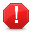# Access UI ComponentThis documentation is for an older version of ZK. For the latest one, please click here.

### Use Java

Every ZUL component has its mapping java class. Every ZUL element declared in ZUML has its mapping java object. You can easily access the object in java code if you assign it an `id`.

The Path class provides utilities to simplify the location of a component among ID spaces. Its usage is similar to `java.io.File`. Like a path in a file system, a component path is a catenation of IDs of components along ID spaces. By default, the root of a component path is the current page. If you want to identify another page, you have to use "//".

Note that, elements that can be space owner (those component class that impelments IdSpace) such as `page`, `window`, `include`, and `regular macro`. (Note: Components on an include-page are not accessible with the same path expression as if they were on the containing page.)

```Path.getComponent("//P/A/B/D");//since C is not a spaceOwner, we dont have to go through C.
Path.getComponent("/A/B/D");//assume the current page is P, then you can omit //P
```

For example, If you have a `label` in ZUML as below,

```<?page id="P"?>
<window id="A">
<window id="B">
<div id="C">
<label id="D"/>
</div>
</window>
</window>
```

then, you can access it as java object by retrieving it through `Path.getComponent`

```Label label = (Label) Path.getComponent("//P/A/B/D");
```

### Use zscript

It's intuitive to access ZUL element in zscript.

```<window>
<label id="lab_1" value="initial by label"/>
<zscript>
lab_1.value = "changed by zscript";
</zscript>
</window>
```

The result will show

```changed by zscript
```

But you have to pay attention to the declaration sequence. Because zscript is evaluated when a ZUML page is rendered. If we adjust the sequence of previous example.

```<window>
<zscript>
lab_1.value = "changed by zscript";
</zscript>
<label id="lab_1" value="initial by label"/>
</window>
```

The result will show

```initial by label
```

Notice the namespace is hierarchical. That means components from upper id space is accessible, unless it's overridden. But components in lower id space is not accessible. Therefore, in the following example `zscript` inside `window win_1_1` can access `lab_1`

```<window id="win_1">
<label id="lab_1" value="initial by label" />
<window id="win_1_1">
<zscript>
lab_1.value = "changed by zscript";
</zscript>
</window>
</window>
```

and the result shows

```changed by zscript
```

If we modify the namespace structure to

```<window id="win_1">
<window id="win_1_1">
<label id="lab_1" value="initial by label" />
</window>
<zscript>
lab_1.value = "changed by zscript";
</zscript>
</window>
```

The result will be

```initial by label
```

Just like Java, you can use `Path.getComponent` in `zscript` for sure.

```<window id="win_1">
<window id="win_1_1">
<label id="lab_1" value="initial by label" />
</window>
<zscript>
public void sayHello()
{
Label l = (Label)Path.getComponent("/win_1/win_1_1/lab_1");
l.setValue("changed");
}
</zscript>
<button label="ok" onClick="sayHello()"/>
</window>
```

In above example, `sayHello()` is evaluated when the `button` is clicked. Therefore, it isn't affected by declaration sequence.

### Use Expression Language

Like `zscript`, EL have to pay attention of declaration sequence. In following example,

```<window>
1:\${btn_1.label}
<button id="btn_1" label="button_1"/>
2:\${btn_1.label}
</window>
```

the result will show,

```1:2:button_1
```

Like `zscript`, the namespace is hierarchical. In the following example,

```<window id="win_1">
<label id="lab_1" value="myValue" />
1:\${lab_1.value}
<window id="win_1_1">
1.1:\${lab_1.value}
</window>
</window>
```

the result will show:

```myValue  1:myValue
1.1:myValue
```

And if we modify the structure,

```<window id="win_1">
<window id="win_1_1">
<label id="lab_1" value="myValue" />
1.1:\${lab_1.value}
</window>
1:\${lab_1.value}
</window>
```

the result will be:

```myValue 1.1:myValue
1:
```

Unlike `zscript`, EL doesn't accept operator "=".

Notes

1. Tip: Path.getComponent("//xyz") always returns null, since the identifier following // is the page's ID. And, page is not component.
2. Typical use of `zscript` includes initialization and declaring global variables and methods.

Last Update : 2022/01/19